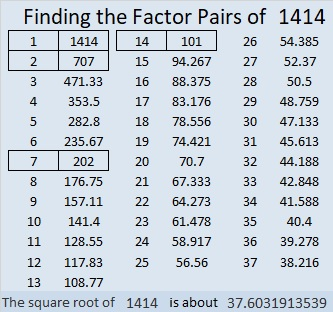# 1414 Your Math Education Post Will Add So Much to This Month’s Carnival!

Have you written a blog post that would bring delight to a preschool, K-12 or homeschool mathematics teacher or student? Then submit it to this month’s Playful Math Education Blog Carnival or message me on Twitter by Friday, September 20th! I’m hosting the carnival this month, and I would love to read your post. So come join the fun!

Today’s puzzle looks a little like a wild, but fun? carnival ride. The numbers 36 and 12 went together on the ride. They managed to stay with each other but the ride went so fast, you can see 36 and 12 in two different places at the same time. There’s also poor number 40. You can see it in THREE places at the same time.

Oh my! Can you use logic to find where the numbers 1 to 10 need to go in both the first column and the top row so that this wild ride will behave like a multiplication table? It’s a level 5 so it won’t be easy to find its unique solution. Are you brave enough to try?Print the puzzles or type the solution in this excel file: 10 Factors 1410-1418

That puzzle’s number is 1414. Let me tell you a little about that number:

• 1414 is a composite number.
• Prime factorization: 1414 = 2 × 7 × 101.
• 1414 has no exponents greater than 1 in its prime factorization, so √1414 cannot be simplified.
• The exponents in the prime factorization are 1, 1, and 1. Adding one to each exponent and multiplying we get (1 + 1)(1 + 1)(1 + 1) = 2 × 2 × 2 = 8. Therefore 1414 has exactly 8 factors.
• The factors of 1414 are outlined with their factor pair partners in the graphic below.1414 is also the hypotenuse of a Pythagorean triple:
280-1386-1414 which is 14 times (20-99-101)